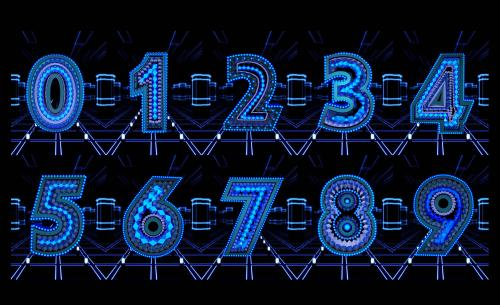# 关注数学发展,弘扬科学精神,专注数学科普

## 从宝塔说到阴阳数串1*8+1=9

12*8+2=98

123*8+3=987

1234*8+4=9876

12345*8+5=98765

123456*8+6=987654

1234567*8+7=9876543

12345678*8+8=98765432123456789*8+9=987654321

9*9+7=88

98*9+6=888

987*9+5=8888

9876*9+4=88888

98765*9+3=888888

987654*9+2=8888888

9876543*9+1=88888888

98765432*9+0=888888888

987654321*9+（-1）=8888888888

9876543210*9+（-2）=88888888888

9876543210（-1）*9+（-3）=8888888888888（声明：本文仅代表作者观点，不代表本站观点，仅做陈列之用）

[责编：大鱼]